Math Concepts

# Integers: Concepts, Properties and Examples

2.4k views

Table of Contents

 1 Introduction 2 What are Integers? 3 Operations of Integers 4 Properties of Integers 5 Application of Integers 6 Summary 7 Frequently Asked Questions (FAQs)

## Introduction

This article will help you learn what integers are, and their use. You will also learn

• Closure property under different operations in integers
• Commutative property under different operations
• Associative property of integers under different operations
• Distributive property of multiplication over addition and subtraction of integers

Also Read,

### Integers: Concepts, Properties and Examples-PDF

This article will help you learn what integers are, and its use. You will also learn the Closure property, Commutative property, Associative property, and Distributive property.  Here is a downloadable PDF to explore more.

 📥 Integers: Concepts, Properties, and Examples-PDF Download

## What are Integers?

A quick look at the numbers before getting to learn what integers are. All digits are grouped together as Numbers. Numbers are classified into various types and a few are listed below,

• Natural Numbers
• Whole Numbers
• Integers, etc.,

Natural Numbers starts from 1 and the series goes endless
Whole Numbers starts with 0 and the series goes endless
Integers comprise the Natural and the Whole numbers. The difference it makes is in holding both positive and negative values.
If Natural Numbers are 1 2 3 4 5 . . .and Whole Numbers are 0 1 2 3 4 5 . . .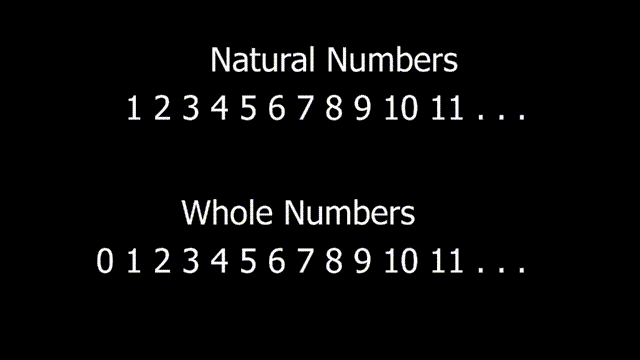Then Integers are. . . -5 -4 -3 -2 -1 0 1 2 3 4 5 . . .
Where 0 being the center of the series and the movement of numbers takes place in both the directions. Moving left from 0 adds a negative sign to numbers while moving right from 0 will add a positive sign to the numbers. In short, a positive number is not denoted with symbol +. The absence of symbol + represents that the number is positive, treated as default.
Example 7, means this number is +7 a positive integer
However, a negative number (-) sign should be placed in front of the number.
Example -7, means the number 7 in a negative value a negative integer, so finds its place towards the left-hand side of 0 in the series.
. . . -7 -6 -5 -4 -3 -2 -1 0 1 2 3 4 5 6 7 . . .
0 is neither positive nor negative integer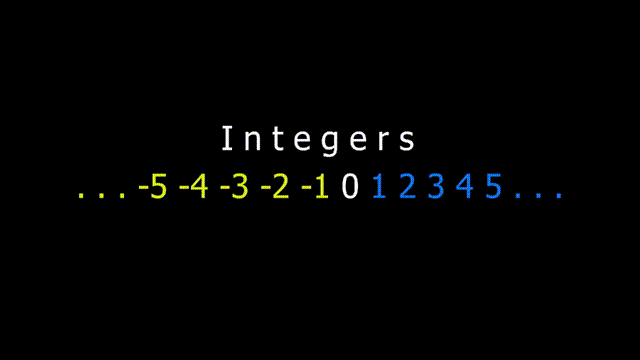The series is consecutive in nature be it towards the right or towards the left.
Towards left means, a series of negative consecutive integers, while towards the right means series of positive consecutive integers.

## Operations of Integers

This session discusses how operations determine the outcome of integer values.
Addition and subtraction of integers using number line and Rules
Follow the below rules to operate on integers

• RULE 1 - Move towards the right, while adding
• RULE 2 - Move towards left, while subtracting

### Illustration 1

-4 + 5 = 1
The above sum is represented using the number line below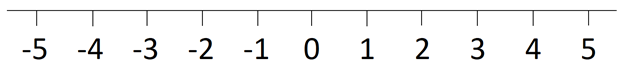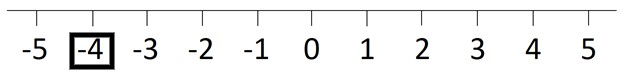(-4) + 5 = ?
The sum represents -4 being ADDED with 5
Rule 1, states that for addition move towards the right
So,
Starting from -4 moving 5 points towards the right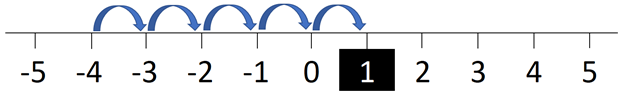(-4) + 5 = 1 which is a positive integer

### Illustration 2

3 – 5 = -2
The above sum is represented using the number line below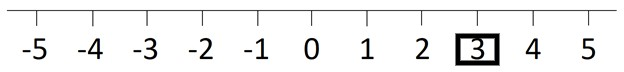3 – 5 = ?
The sum represents (-5) being SUBTRACTED from 3
Rule 2, states that for subtraction, move towards left
So,
Starting from 3 moving 5 points towards left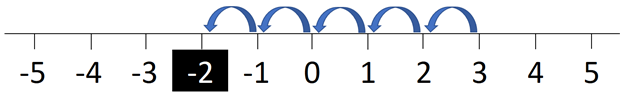3 – 5 = -2 which is a negative integer

Moving on, the idea of a number line for larger numbers becomes impossible to use.
So memorize the below rules,

• RULE 3 - If two numbers have the same sign, add them and keep the sign
• RULE 4 - If two numbers have different signs, subtract and use the sign of the larger number

### Illustration 3

24 + 56 = ?
24 is a positive integer
56 is also a positive integer
RULE 3, states that if 2 integers have the same sign, then add and keep the sign
(+24) + (+56) = +80

### Illustration 4

-24 + -56 = ?
24 is a negative integer
56 is a negative integer
RULE 3, states that if 2 integers have the same sign, then add and keep the sign
(-24) + (-56) = -80

### Illustration 5

24 - 56 = ?
24 is a positive integer
56 is also a negative integer
RULE 4, states that If two numbers have different signs, subtract and use the sign of the larger number
24 - 56 = -32
Subtraction value is 32
-  sign, because the larger value from 24 and 56 is 56. Hence the result should carry - sign as per RULE 4
Answer is -32

### Illustration 6

-24 + 56 = ?
24 is a negative integer
56 is also a positive integer
RULE 4, states that If two numbers have different signs, subtract and use the sign of the larger number
(-24) + 56 = 32
Subtraction value is 32
+  sign, because the larger value from 24 and 56 is 56. Hence the result should carry + sign as per RULE 4
The answer is +32, in other forms written as 32.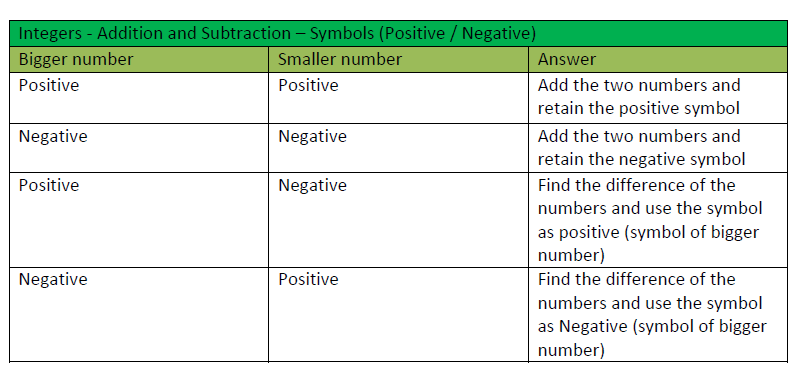## Multiplication and division of integers using Rules

• RULE 5 - When you multiply or divide 2 numbers having the same sign, the answer is positive
• RULE 6 - When you multiply or divide 2 numbers having different signs, the answer is negative

NOTE - Do not confuse with rules of addition and subtraction with multiplication and division

30 x 2 = ?
Apply Rule 5
30 x 2 = 60

- 30 x 2 = ?
Apply Rule 6
30 x 2 = -60

30 ÷ 2 =?
Apply Rule 5
30 ÷ 2 = 15

30 ÷ (-2) = ?
Apply Rule 6
30 ÷ (-2) = -15

## Exponents on negative integers

• Rule 7 - Even exponent on a negative number gives a positive answer
• Rule 8 - Odd exponent on a negative number gives a negative answer

(-5)2 = ?
Apply Rule 7
-5 x -5 = 25

### Illustration 12

(-5)3 = ?
Apply Rule 8
(-5) x (-5) x (-5)

Step 1
(-5) x (-5) x (-5)
25      x (-5)

Step 2
25 x (-5)
-125

Answer (-5)3 = -125

## Properties of Integers

This session will discuss the change in properties of the integers w.r.t operators used

### Closure property

Closure property means, when an operator is used in between integers and the resulting answer is also an integer, the property is said to be closed when that particular operator is used.

But the closure property holds good only for certain operators. We will look into detail about this property below: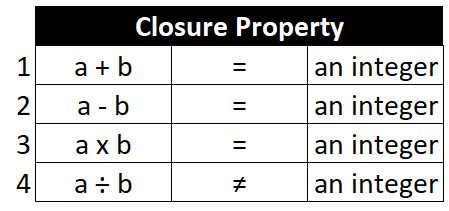Where a and b are 2 integers

### Illustration 13

a + b = an integer
If a is 3 and b is 5
Then, a + b = 3 + 5 = 8
8 is an integer

### Illustration 14

a + b = an integer
If a is -3 and b is 5
Then, a + b = -3 + 5 = 2
2 is an integer

### Illustration 15

a - b = an integer
If a is 3 and b is 5
Then, a - b = 3 - 5 = -2
-2 is an integer

### Illustration 16

a - b = an integer
If a is -3 and b is - 5
Then, a - b = (-3) -(- 5) = 2 (RULE 3)
2 is an integer

### Illustration 17

a x b = an integer
If a is 3 and b is 5
Then, a x b = 3 x 5 = 15
15 is an integer

### Illustration 18

a x b = an integer
If a is 3 and b is -5
Then, a x b = 3 x (-5) = -15 (RULE 6)
-15 is an integer

### Illustration 19

a ÷ b ≠ an integer
If a is 4 and b is 2
Then, a ÷ b = 4 ÷ 2 = 2
However, if a is 2 and b is 4
Then, a ÷ b = 2 ÷ 4 = 1/2
1/2 is not an integer
So, not all the integers when divided will result as an integer.
Hence, Division is not a closed property of Integer.

### Learning outcome

• Integers are closed under Addition, Subtraction, and Multiplication.
• Integers are not closed under Division.

### Commutative property

The commutative property, as the name suggests (commute ~ commutative), we could commute in any direction. Commutative property means when an operator is used between two numbers or integers, the final answer should be the same when worked either way, from left to right or right to left.

But, the commutative property holds good for only certain operators and the details are given below,Where a and b are 2 integers

### Illustration 20

a + b = b + a
If a is 4 and b is 2
Then,
a + b = b + a
4 + 2 = 2 + 4
6   =    6
Addition is commutative for integers

### Illustration 21

a - b ≠ b - a
If a is 4 and b is 2
Then,
a - b ≠ b - a
4 - 2 ≠ 2 - 4
2   ≠    -2
Subtraction is not commutative for integers

### Illustration 22

a x b = b x a
If a is 4 and b is 2
Then,
a x b = b x a
4 x 2 = 2 x 4
8    =    8
Multiplication is commutative for integers

### Illustration 23

a ÷ b ≠ b ÷ a
If a is 4 and b is 2
Then,
a ÷ b ≠ b ÷ a
4 ÷ 2 ≠ 2 ÷ 4
2   ≠    1/2
The division is not commutative for integers

### Learning outcome

• Addition and Multiplication are commutative for Integers
• Subtraction and Division are not commutative for Integers

### Associative property

The associative property, as the name suggests (Associative ~ Association) means that the grouping of terms will not affect the final answer when grouped in different ways.

But the Associative property holds good only for certain operators. The details are given below: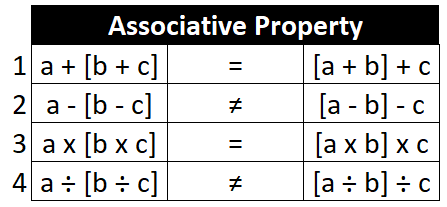Where a b c are integers

The distributive property is applicable

1. When a group involving the addition of 2 integers is multiplied by another integer. Here it involves 2 operations. Addition and Multiplication. It indicates that we can either add first and then multiply or Multiply first and then add next.
2. When a group involving the subtraction of 2 integers is multiplied by another integer. So it involves 2 operations. Subtraction and Multiplication. It indicates that we can either subtract first and then multiply or Multiply first and then subtract next.

### Illustration 24

a + [ b + c ] = [ a + b ] + c
If a is 6, b is 4 and c is 2
Then,
a + [ b + c ] = [ a + b ] + c
6 + [ 4 + 2 ] = [ 6 + 4 ] + 2
6    +    6     =     10  +   2
12       =       12
Addition property is associative for integers

### Illustration 25

a - [ b - c ] ≠ [ a - b ] - c
If a is 6, b is 4 and c is 2
Then,
a - [ b - c ] ≠ [ a - b ] - c
6 - [ 4 - 2 ] ≠ [ 6 - 4 ] - 2
6    -   2     ≠      2  -  2
4       ≠       0
Subtraction property is not associative for integers

### Illustration 26

a x [ b x c ] = [ a x b ] x c
If a is 6, b is 4 and c is 2
Then,
a x [ b x c ] = [ a x b ] x c
6 x [ 4 x 2 ] = [ 6 x 4 ] x 2
6    x    8     =     24  x   2
48       =       48
Multiplication property is associative for integers

### Illustration 27

a ÷ [ b ÷ c ] ≠ [ a ÷ b ] ÷ c
If a is -8, b is 4 and c is -2
Then,
[(-8) / 4] / -2 = -8 / [(4) / (-2)]
a ÷ [ b ÷ c ] ≠ [ a ÷ b ] ÷ c
-8 ÷ [(4) ÷ (-2)] ≠ [ (-8) ÷ 4 ] ÷ (-2)
-8     ÷   (-2)     ≠      (-2)  ÷  (-2)
4        ≠       1
Division property is not associative for integers

### Learning outcome

• Addition and Multiplication are Associative for Integers
• Subtraction and Division are Not Associative for Integers

### Distributive property

As the name (distributive ~ distribution) indicates, a factor or a number or an integer along with the operation multiplication (‘x’),  is getting distributed to the numbers separated by either addition or subtraction inside the parenthesis.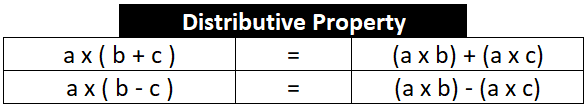Where a b and c are integers

The distributive property is applicable when a group involving the addition of 2 integers is multiplied by another integer. So it involves 2 operations. Addition and multiplication.
It indicates that we can either add first and then multiply or Multiply first and then add next.

### Illustration 28

a x ( b + c ) = ( a x b ) + ( a x c )
If a is -2, b is 4 and c is 3
Then,
a x ( b + c ) = ( a x b ) + ( a x c ) is
-2 x (4 + 3) = [(-2) x 4] + [(-2) x 3]
(-2) x 7 = (-8) + (-6)
-14 = -14

### Illustration 29

a x ( b - c ) = ( a x b ) - ( a x c )
If a is 4, b is 3 and c is 6
Then,
a x ( b - c ) = ( a x b ) - ( a x c ) is
4 x (3 – 6) = 4 x 3 – 4 x 6
4 x -3 = 12 - 24
-12 = -12
Multiplication is a distributive property of integers.

### Learning outcome

• Multiplication is distributive over addition for Integers
• Multiplication is distributive over subtraction for Integers

## Application of Integers

### Illustration 30

Elevator or Lifts we use in daily life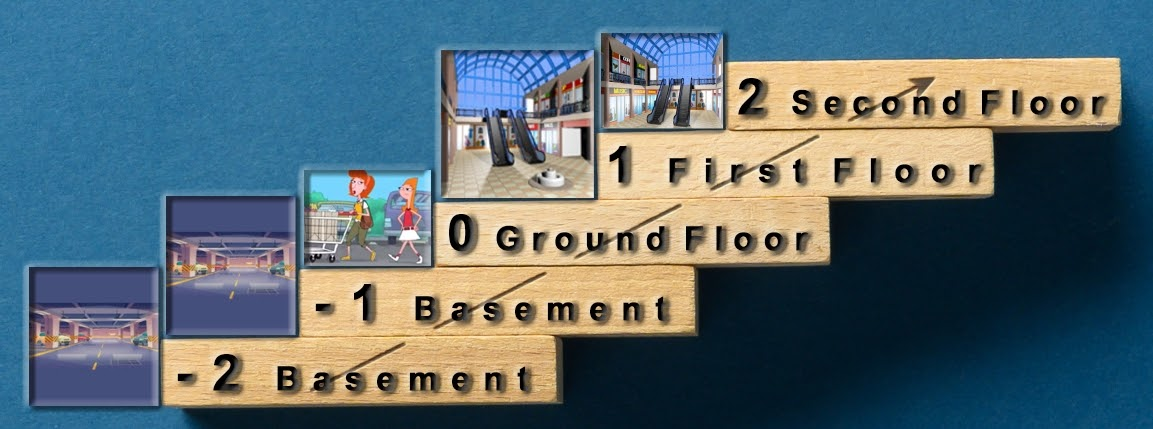### Illustration 31

Atmospheric Temperature
Temperatures of countries differ based on geographic locations.
For instance, Greenland recorded the coldest temperature of -66 °C and the hottest temperature of 30 °C depending upon the season.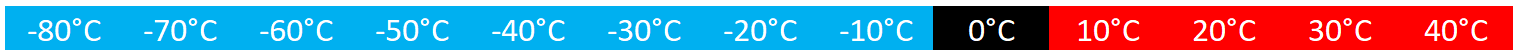### Illustration 32

Usage of positive and negative integers in coding
Int main ()
{
int a;
printf (“Enter the integer”);
scanf (“%d”, &a );

If (a > 0)
printf (“%d is a positive integer”, a);
elseif (a < 0)
printf (“%d is a negative integer”, a);
elseif (a == 0)
printf (“%d is zero”, a);
return 0;
}

Output
Run 1
Enter the integer = 10
10 is a positive integer

Run 2
Enter the integer = -10
-10 is a negative integer

Run 3
Enter the integer = 0
0 is zero

## Summary

Usage of symbols in the problems with Integers can be easily remembered with the below table: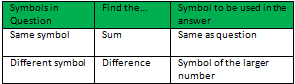The properties of integers explained in this document are summarised in the below table. This can be used as a reference while practising integers.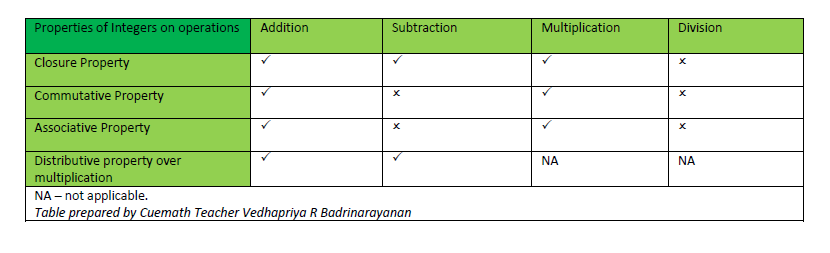## What is an integer?

An integer is a series of numbers that range from -∞ (infinity) to +∞ (infinity) without decimal values.

## Are Even numbers Integers?

All even numbers are integers.

## Are Odd numbers Integers?

All odd numbers are integers.

## How do you identify, if a number is an Integer or not?

An integer is any number that has no decimals, though it may still be either positive or negative in nature.

## Is Zero an Integer?

Yes. Zero falls between -∞ (infinity) to +∞ (infinity) and hence it is an integer, though it neither carries a positive (+) or a negative (-) sign.

## Is Zero positive or a negative Integer?

Though Zero is an integer, it neither carries a positive (+) or a negative (-) sign. Any number added or subtracted with Zero gives the same number as result, and hence the sign for Zero is ignored or not considered.

## External References

-Written by Vishali B, Cuemath Teacher

Related Articles
GIVE YOUR CHILD THE CUEMATH EDGE
Access Personalised Math learning through interactive worksheets, gamified concepts and grade-wise courses
Learn More About Cuemath